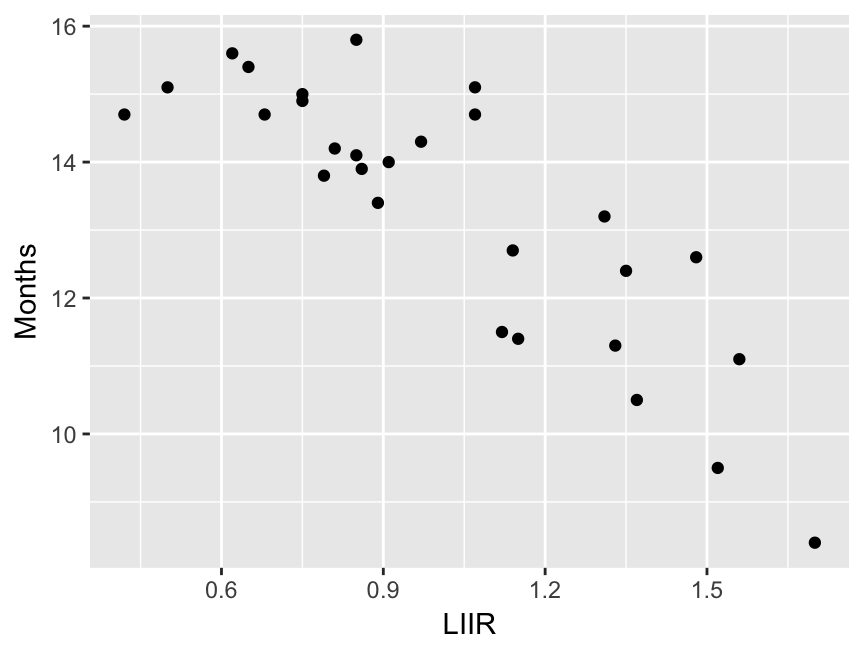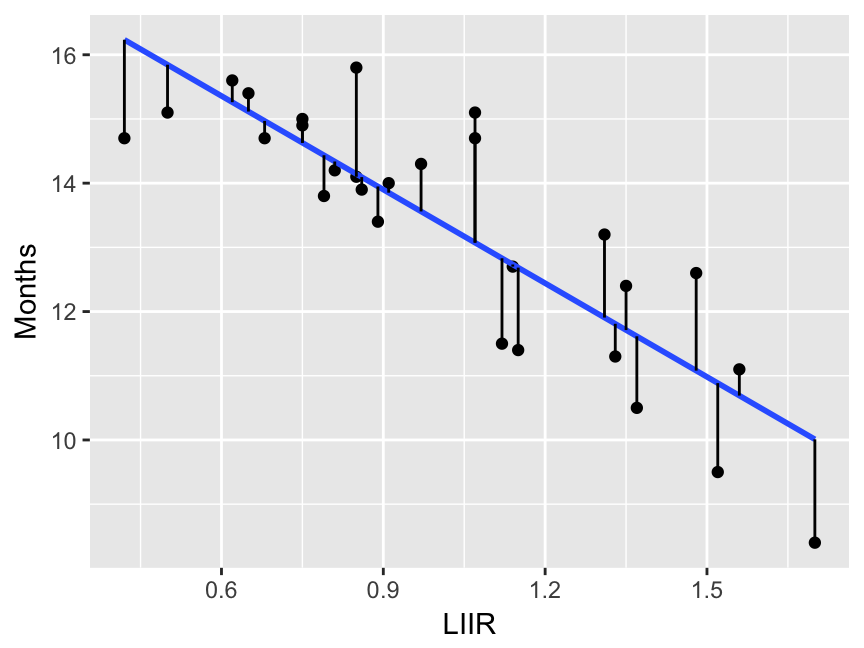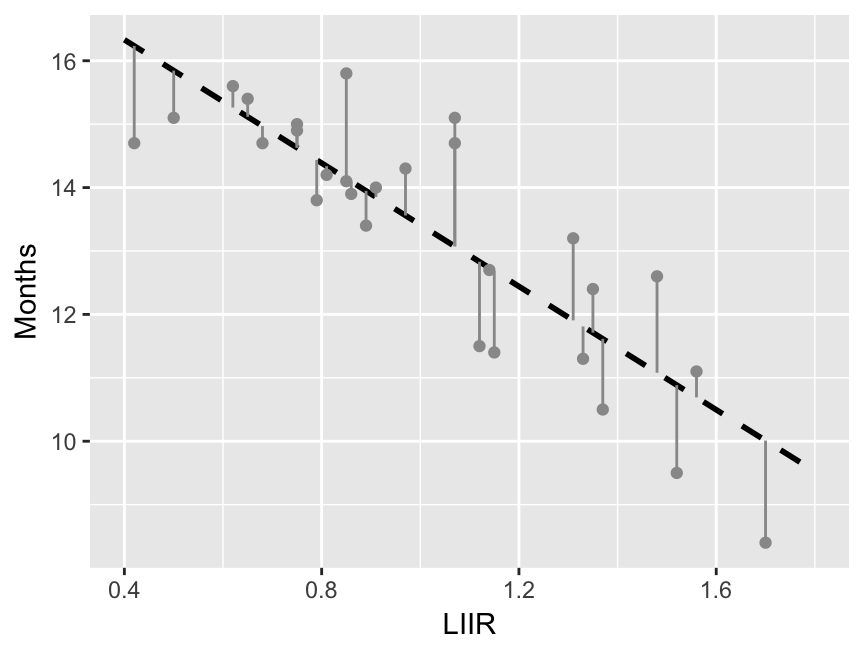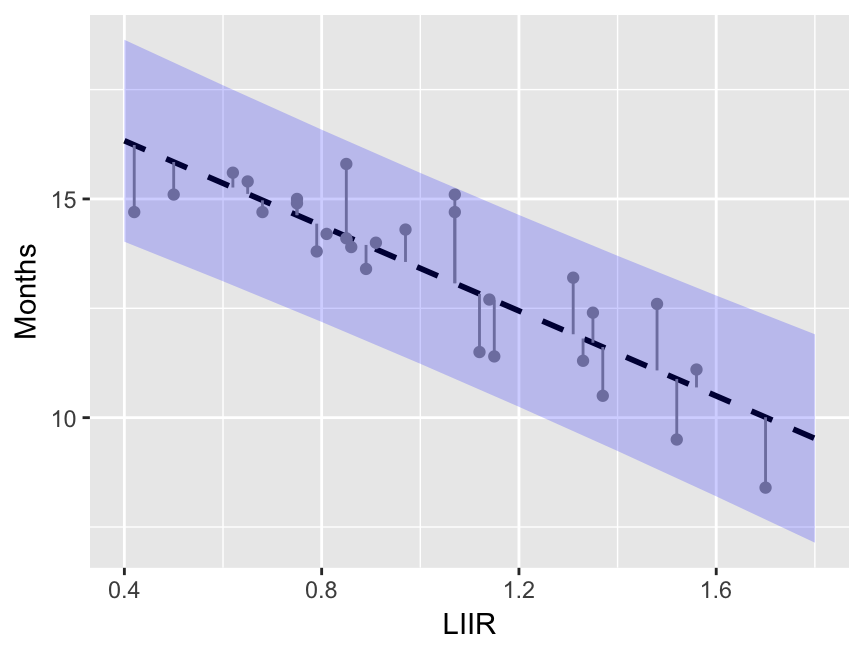# Predicting duration before credit default of borrowers based on loan-installment-to-income ratio

This post is a short demonstration of a simple regression analysis based on a mock data set (`dat`) that consists of loan-installment-to-income ratios of 28 borrowers (`LIIR`) and the number of months before default (`Months`). I want to revise the basic steps for regression analysis in R and would like to show practitioners that error in machine – or statistical – learning is unavoidable.

``````library(tidyverse)
``````## # A tibble: 28 x 2
##     LIIR Months
##    <dbl>  <dbl>
##  1  0.85   15.8
##  2  1.70    8.4
##  3  1.37   10.5
##  4  0.50   15.1
##  5  1.52    9.5
##  6  1.12   11.5
##  7  0.62   15.6
##  8  0.89   13.4
##  9  0.68   14.7
## 10  1.48   12.6
## # ... with 18 more rows``````

This is a basic excercise in modelling and prediction using R and it is based on a data set consisting of two columns for defaulted borrowers whose loan-installment-to-income ratios – `LIIR` – had been recorded `Months` before: given the LIIR ratio we want to predict how many months it takes before the borrower defaults.

## Regression analysis for modelling data

“One should always plot the data first, before any model is fit to them” I have been reminded time and again first as a student and then as a teacher (by my students!) to check for heteroskedasticity, for instance.

``````dat %>%
ggplot(aes(LIIR, Months)) +
geom_point()``````The data set

A rough plot of our data shows a strong linear (?) pattern that can be fit with a linear model.

``dat_mod <- lm(Months ~ LIIR, data = dat)``
``````sm <- summary(dat_mod)
sm``````
``````##
## Call:
## lm(formula = Months ~ LIIR, data = dat)
##
## Residuals:
##      Min       1Q   Median       3Q      Max
## -1.61030 -0.66229 -0.03807  0.47875  2.02659
##
## Coefficients:
##             Estimate Std. Error t value Pr(>|t|)
## (Intercept)  18.2758     0.6361  28.731  < 2e-16 ***
## LIIR         -4.8621     0.5948  -8.174 1.18e-08 ***
## ---
## Signif. codes:  0 '***' 0.001 '**' 0.01 '*' 0.05 '.' 0.1 ' ' 1
##
## Residual standard error: 1.043 on 26 degrees of freedom
## Multiple R-squared:  0.7199, Adjusted R-squared:  0.7091
## F-statistic: 66.81 on 1 and 26 DF,  p-value: 1.179e-08``````

Check the overall significance by looking at the F-statistic at the bottom of the summary report, in this case its p-value is 1.179e-08 meaning this model does a better job with these data in predicting the response variable rather than its mean value (the mean is the most basic model and in this case the predicted response based on the mean would be 13 months – the average of `Months` in the data set). Take a look at the R-squared: 0.72 of the variance in these data is accounted for by the model.

I’ll plot the model – and its residuals – using the outcome generated by `stat_smooth`(which is the same smoother line as the one fitted by us before).

``````ggplot(dat, aes(LIIR, Months)) +
geom_point() +
stat_smooth(method = "lm",
se = FALSE) +
geom_segment(aes(xend = LIIR, yend = predict(dat_mod)))``````Here I want to follow the marks of R for data science of Garrett Grolemund and Hadley Wickham by creating a data grid using the `modelr` package and then adding the predictions to it.

``````library(modelr)
grd <- data_grid(dat, LIIR = seq_range(LIIR, n = 8, pretty = TRUE))
grd <- add_predictions(grd, dat_mod, var = "Months")
grd``````
``````## # A tibble: 8 x 2
##    LIIR    Months
##   <dbl>     <dbl>
## 1   0.4 16.331003
## 2   0.6 15.358587
## 3   0.8 14.386172
## 4   1.0 13.413756
## 5   1.2 12.441341
## 6   1.4 11.468925
## 7   1.6 10.496510
## 8   1.8  9.524094``````

When the LIIR ratio is 0.4 we expect 16.3 months before default and if the LIIR ratio increases to 1 we forecast 13.4 months before default.

Let’s plot the predictions generated by our linear model together with the residuals to remind us of the error implicit in any forecast.

``````pl_p <- ggplot(grd, aes(x = LIIR, y = Months)) +
geom_line(size = 1.0, linetype = "dashed") +
geom_point(data = dat, color = "gray60") +
geom_segment(data = dat, aes(xend = LIIR, yend = predict(dat_mod)), color = "gray60")
pl_p``````If I want to make a prediction interval using the LIIR in data grid I should proceed as follows.

``````pred <- as.tibble(predict(dat_mod, newdata = grd, interval = "predict"))
pred <- pred %>% mutate(LIIR = grd\$LIIR)
pred``````
``````## # A tibble: 8 x 4
##         fit       lwr      upr  LIIR
##       <dbl>     <dbl>    <dbl> <dbl>
## 1 16.331003 14.022751 18.63926   0.4
## 2 15.358587 13.118275 17.59890   0.6
## 3 14.386172 12.188544 16.58380   0.8
## 4 13.413756 11.232075 15.59544   1.0
## 5 12.441341 10.248285 14.63440   1.2
## 6 11.468925  9.237591 13.70026   1.4
## 7 10.496510  8.201340 12.79168   1.6
## 8  9.524094  7.141584 11.90660   1.8``````

Now, I want to plot the point estimate together with the prediction interval.

``````pl_p + geom_ribbon(data = pred, aes(ymin = lwr, ymax = upr,
x = LIIR, y = fit),
fill = "blue", alpha = 0.2)``````Posted in Economics, R-stats
Tags: ,

### Tweets

Error: Could not authenticate you.